American Journal of Applied Mathematics
Volume 4, Issue 5, October 2016, Pages: 217-221

The Solution of Porous Medium Equation by Aboodh Homotopy Perturbation Method

Mohand M. AbdelrahimMahgoub1, 2, Abdelilah K. Hassan Sedeeg1, 3

1Mathematics Department Faculty of Sciences and Arts Almikwah, Albaha University, Albaha, Saudi Arabia

2Mathematics Department Faculty of Sciences-Omdurman Islamic University, Khartoum, Sudan

3Mathematics Department Faculty of Education- Holy Quran and Islamic Sciences University, Khartoum, Sudan

Email address: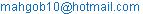(M. M. A. Mahgoub)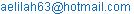(A. K. H. Sedeeg)

To cite this article:

Mohand M. Abdelrahim Mahgoub, Abdelilah K. Hassan Sedeeg. The Solution of Porous Medium Equationby Aboodh Homotopy Perturbation Method. American Journal of Applied Mathematics. Vol. 4, No. 5, 2016, pp. 217-221. doi: 10.11648/j.ajam.20160405.13

Received: August 26, 2016; Accepted: September 3, 2016; Published: October 9, 2016

Abstract: In this paper, the homotopy perturbation method (HPM) and Aboodh transform are employed to obtain analytical solution of the porous medium equation. The proposed method (ATHPM) is an elegant combination of the new integral transform "Aboodh Transform" and the homotopy perturbation method. The porous medium equations have importance in engineering and sciences and constitute a good model for many systems in various fields. The results tell us that the proposed method is more efficient and easier to handle when is compared with existing other methods in such partial differential equations.

Keywords: Aboodh Transform, Homotopy Perturbation Method, Non Linear Partial Differential Equation, Porous Medium Equation

Contents

1. Introduction

The heat equation is one of the three classical linear partial differential equations of second order that form the basis of any elementary introduction to the area of PDEs, and only recently has it come to be fairly well understood. The nonlinear heat equation usually called the Porous Medium Equation [5-7] (PME).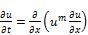(1)

where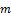is a rational number.

This equation appears in a number of physical applications, such as to describe processes involving fluid flow, heat transfer, or diffusion. Other applications have been proposed in mathematical biology, lubrication, boundary layer theory, and other fields. All of these reasons support the interest of its study both for the mathematician and the scientist. In the resent years, many researchers mainly had paid attention to studying the solution of nonlinear partial differential equations by using various methods. Among these are the variational iteration method [Biazar and Ghazvini (2007)] , Adomian decomposition method [Wazwaz (2001) and Biazar et al (2007)], homotopy perturbation method [Mehdi Dehghan and JalilManafian (2008)], homotopy analysis method [Najeeb Alam Khan, Asmat Ara, Muhammad Afzal and Azam Khan (2010)], Elzaki Homotopy Perturbation Method [8-10], and Laplace decomposition algorithm [Majid Khan, Muhammad Asif Gondal and Yasir Khan (2011)]. Motivated and inspired by the on-going research inthese areas, we consider a new method, which is called Aboodh transform homotopy perturbation method (ATHPM) [1-4]. This method is suggested by combining the homotopy perturbation method and Aboodh transform. This method is a useful technique for solving linear and nonlinear differential equations. This article considers the effectiveness of the homotopy perturbation Aboodh transform method in solving. The porous medium equation.

2. Aboodh Transform Homotopy Perturbation Method

The general form of nonlinear non homogeneous partial differential equation can be considered as the follow: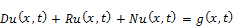(2)

with the following initial conditions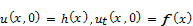Where D is the second order linear differential operator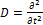, is the linear differential operator of less order than D, N represents the general non-linear differential operator and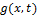is the source term.

Ting Aboodh transform (denoted throughout this paper by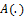) on both sides of Eq. (2), to get: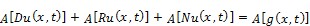(3)

Using the differentiation property of Aboodh transform and above initial conditions, we have: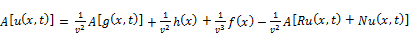(4)

Operating with the Aboodh inverse on both sides of Eq. (4) gives: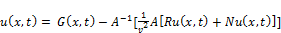(5)

Where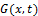represents the term arising from the source term and the prescribed initial condition.

Now, we apply the homotopy perturbation method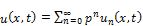(6)

And the nonlinear term can be decomposed as: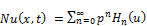(7)

Where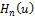are He’s polynomial and given by: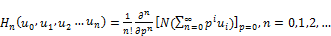(8)

Substituting Eqs. (6) and (7) in Eq. (5) we get: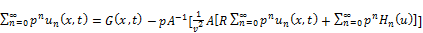(9)

Which is the coupling of the Aboodh transform and the homotopy perturbation method using He’s polynomials. Comparing the coefficient of like powers of, the following approximations are obtained: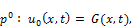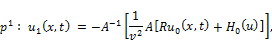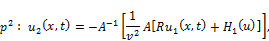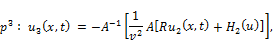... ... ... ... ... ... ... ... ...

Then the solution is;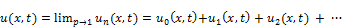(10)

3. Applications

In this section we apply the homotopy perturbation Aboodh transform method for solving the porous medium equation.

Example 1:

Let us take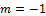in equation (1), we get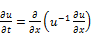(11)

With the initial condition;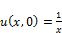(12)

Exact solution  of this equation is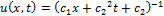with the values of arbitrary constants taken as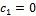and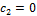, thusthe solution becomes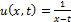.

We can find solution by applying the Aboodh transform of both sides of Eq. (11) subject to the initial condition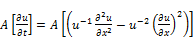(13)

Using the differential property of Aboodh transform Eq. (13) can be written as: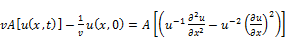(14)

Using initial condition Eq. (12), Eq. (14) can be written as: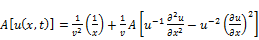(15)

The inverse Aboodh transform implies that: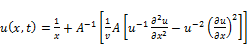(16)

Now, we apply the homotopy perturbation method, we get: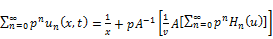(17)

Where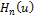are He's polynomial s. The first two components of He's polynomials are given by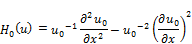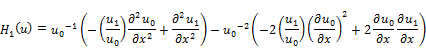Comparing the coefficient of like powers of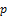, the following approximations are obtained;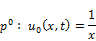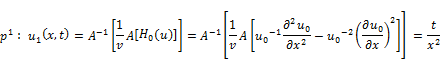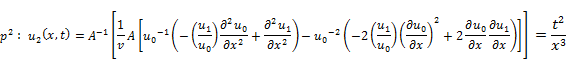Proceeding in similar manner we can obtain further values, substituting above values in equation (10), we get solution in the form of a series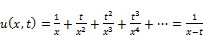(18)

This is the solution of (11) and which is exactly the exact solution given above.

Example 2:

Let us take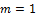in equation (1), we get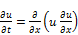(19)

With the initial condition as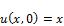(20)

Exact solution  of this equation is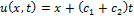with the values of arbitrary constants taken as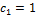and, thusthe solution becomes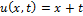.

We can find solution by applying the Aboodh transform of both sides of Eq. (19) subject to the initial condition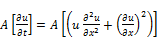(21)

Using the differential property of Aboodh transform Eq.(21) can be written as: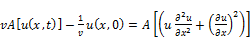(22)

Using initial condition Eq. (20), Eq. (22) can be written as: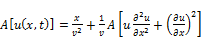(23)

The inverse Aboodh transform implies that: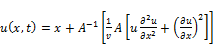(24)

Now, we apply the homotopy perturbation method, we get: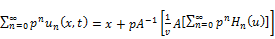(25)

Whereare He's polynomial s. The first two components of He's polynomials are given by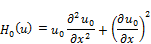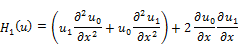Comparing the coefficient of like powers ofin Eq.(25), the following approximations are obtained;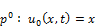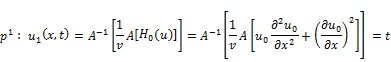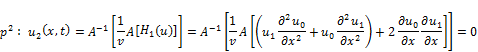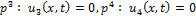and so on we will found that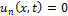for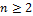.

Substituting above values in equation (10) we get solution in the form of a series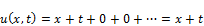(26)

This is the solution of Eq. (19) and which is exactly the exact solution given above.

Example 3:

Let us take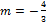in equation (1), we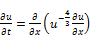(27)

With the initial condition as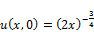(28)

We can find solution by applying the Aboodh transform of both sides of Eq. (27) subject to the initial condition(29)

Using the differential property of Aboodh transform Eq. (21) can be written as: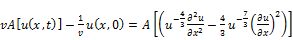(30)

Using initial condition Eq. (20), Eq. (22) can be written as: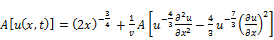(31)

The inverse Aboodh transform implies that: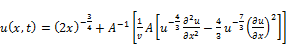(32)

Now, we apply the homotopy perturbation method, we get: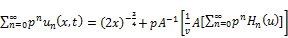(33)

Whereare He's polynomial s. The first two components of He's polynomials are given by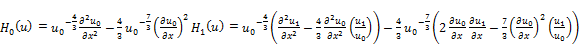(34)

Comparing the coefficient of like powers ofin Eq. (25), the following approximations are obtained;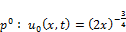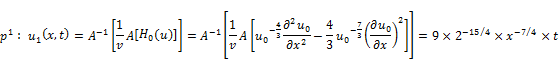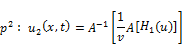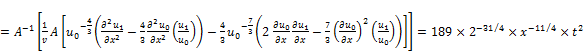(35)

On substituting these terms in equation (10) we obtained solution in the form of a series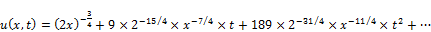(36)

This result can be verified through substitution.

4. Conclusion

In this paper, the ATHPM has been successfully applied to find the solution of the analytical solution for porous medium equation. The method is reliable and easy to use. The results show that the ATHPM is a powerful and efficient technique in finding exact and approximate solutions of the porous medium equation. the method is trustworthy and introduces a significant improvement in solving nonlinear partial differential equations over existing methods.

References

1. K. S. Aboodh, The New Integral Transform "Aboodh Transform" Global Journal of pure and Applied Mathematics, 9(1), 35-43(2013).
2. K. S. Aboodh, Application of New Transform "Aboodh transform" to Partial Differential Equations, Global Journal of pure and Applied Math, 10(2),249-254(2014).
3. Khalid Suliman Aboodh,Homotopy Perturbation Method and Aboodh Transform for Solving Nonlinear Partial Differential Equations, Pure and Applied Mathematics JournalVolume 4, Issue 5, October 2015, Pages: 219-224.
4. Khalid Suliman Aboodh, Solving Fourth Order Parabolic PDE with Variable Coefficients Using Aboodh Transform Homotopy Perturbation Method, Pure and Applied Mathematics Journal 2015; 4(5): 219-224.
5. Mishra D, Pradhan V. H., Mehta M. N. (2012), Solution of Porous Medium Equation by Homotopy Perturbation Transform Method, International Journal of Engineering Research and Applications, Vol.2 Issue 3, pp2041-2046.
6. Juan Luis Vazquez (2007), The Porous Medium Equation Mathematical Theory, Oxford Science Publication, Clarenden Press, pp1-28.
7. PremKiran G. Bhadane1, V. H. Pradhan, Elzaki Transform Homotopy Perturbation Method For Solving Porous Medium Equation, international journal of research in ingineering and technology, pissn: 2321-7308.
8. Tarig M. Elzaki and Eman M. A. Hilal (2012), Homotopy Perturbation and ELzaki Transform for solving Nonlinear Partial Differential equations, Mathematical Theory and Modeling, Vol.2,No.3, pp33-42.
9. Tarig M. Elzaki and Salih M. Elzaki (2011), Applications of New Transform "ELzakiTransform" to Partial Differential Equations, Global Journal of Pure and Applied Mathematics, Vol.7, No.1,pp65-70.
10. Tarig M. Elzaki (2011), The New Integral Transform "ELzaki Transform", Global Journal of Pure and Applied Mathematics, Vol.7, No.1,pp5764.
11. Biazar, J., Ghazvini, H., 2007. Exact solutions for nonlinear Schrodinger equations by He’s homotopy perturbation method. Physics Letter A 366, 79-84.

 Contents 1. 2. 3. 4.
Article ToolsAbstractPDF(174K)
Follow on us
PUBLICATION SERVICE
JOIN US
RESOURCES
SPECIAL SERVICES
ADDRESS
Science Publishing Group
548 FASHION AVENUE
NEW YORK, NY 10018
U.S.A.
Tel: (001)347-688-8931